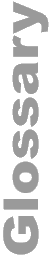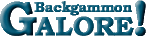Janowski's FormulasJanowski's Formulas A collection of formulas devised by Rick Janowski for estimating cubeful equity from cubeless equity. The basic formula for cubeful equity (between take points) is: ` CF = CL*(1 - x) + CE*x` where CF is cubeful equity, CL is cubeless equity, CE is cubeful equity assuming all doubles are perfectly efficient, and x is a number between 0 and 1 that measures the cube efficiency. Typical values for x range from 0.55 to 0.8.   See also: Janowski's Takepoint Formula.

 Help   •   About the Glossary   •   List of Terms   •   Full Glossary